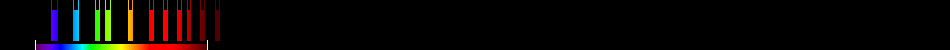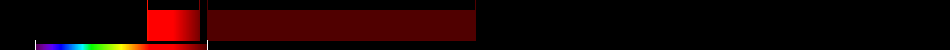# Information for Sensor and Index

## Formula

General Formula $\frac{{\mathrm{NIR}}}{{\mathrm{RED}}+\frac{{b}}{{a}}}$ $\frac{{\mathrm{11}}}{{8}+\frac{{b}}{{a}}}$ Automatic

## Sensor

Name CASI_spatial 11 417-806 0-0 0 airborne ITRES Research Instruments 1989-00-00 Pixel per line: 512, FOV: 34,2Â° Spatial Mode = 512 pixels across swath, up to 18 spectral bands (fully programmable) yes

## Index

Name Soil-adjusted vegetation index 2 SAVI2 $\frac{{\mathrm{NIR}}}{{\mathrm{RED}}+\frac{{b}}{{a}}}$ The actual gain (a) and intercept (b) values of the soil line rather than assuming them to be 1 and 0, respectively 640:760,780:1400 Original formula

## References